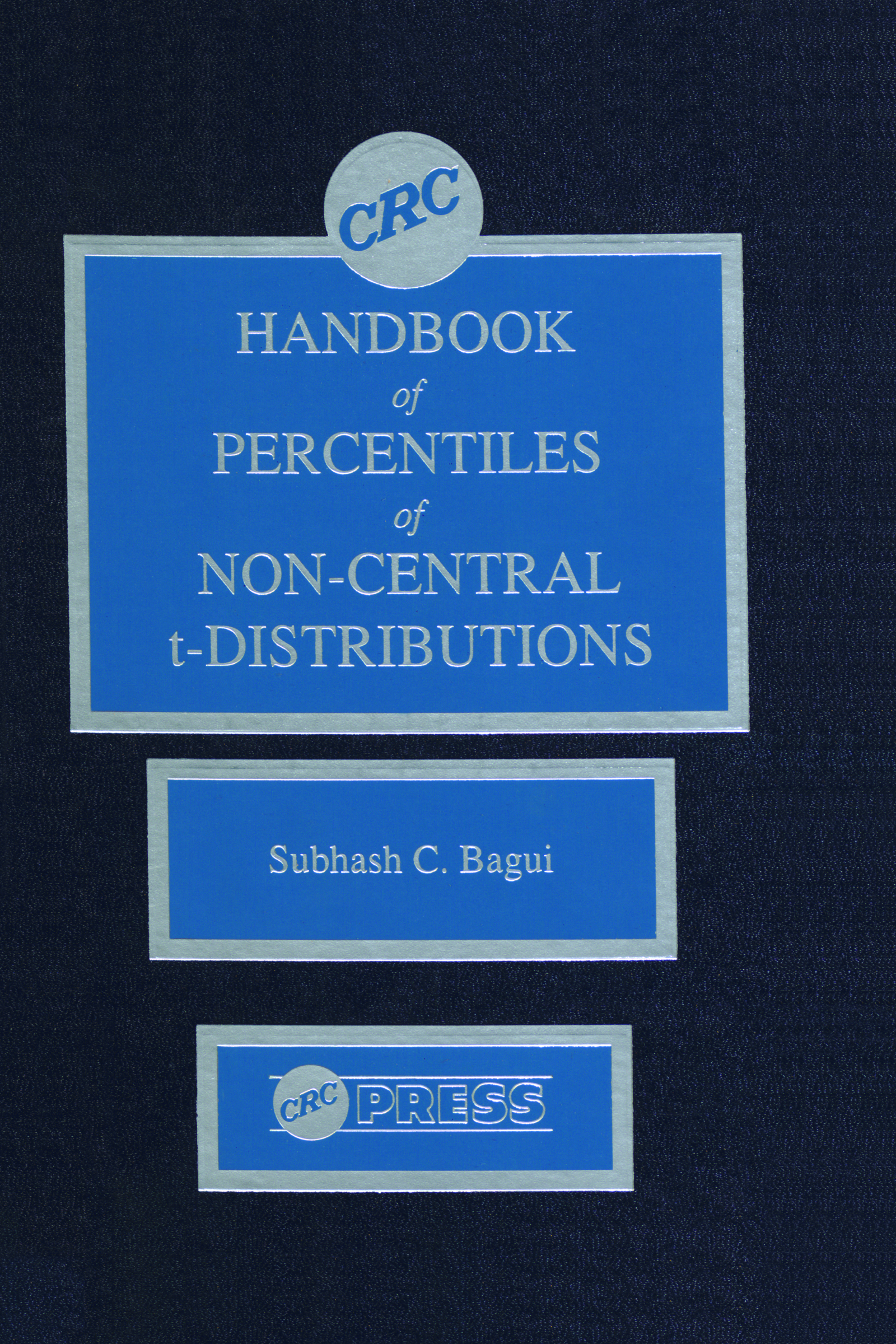# CRC Handbook of Percentiles of Non-Central t-Distributions

## 1st Edition

CRC Press

400 pages

##### Purchasing Options:\$ = USD
Hardback: 9780849386695
pub: 1993-01-13
SAVE ~\$86.00
Currently out of stock
\$430.00
\$344.00
x

FREE Standard Shipping!

### Description

CRC Handbook of Percentiles of Non-Central t-Distributions is the first book to provide critical values of non-central t-distributions in an easy-to-use format. The book presents a brief introductory section that outlines properties and applications of non-central t-distributions and then explains the tables. The rest of the book consists of tables. The values listed were produced using cumulative probabilities.

CRC Handbook of Percentiles of Non-Central t-Distributions is an essential reference of numerical tables of statistical functions for researchers, practitioners, scientists, and students involved with statistics in the areas of tolerance limits, variable sampling plans, confidence limits on quantiles, confidence limits on proportions, sample coefficient of variation and in computing the power of t-test.

### Table of Contents

Introduction: The Critical Values of Non-Central t Distributions. a=0.01, a=0.025, a=0.05, a=0.10, a=0.20, a=0.30, a=0.70, a=0.80, a=0.90, a=0.95, a=0.975, a=0.99. References.

### Subject Categories

##### BISAC Subject Codes/Headings:
MAT029000
MATHEMATICS / Probability & Statistics / General